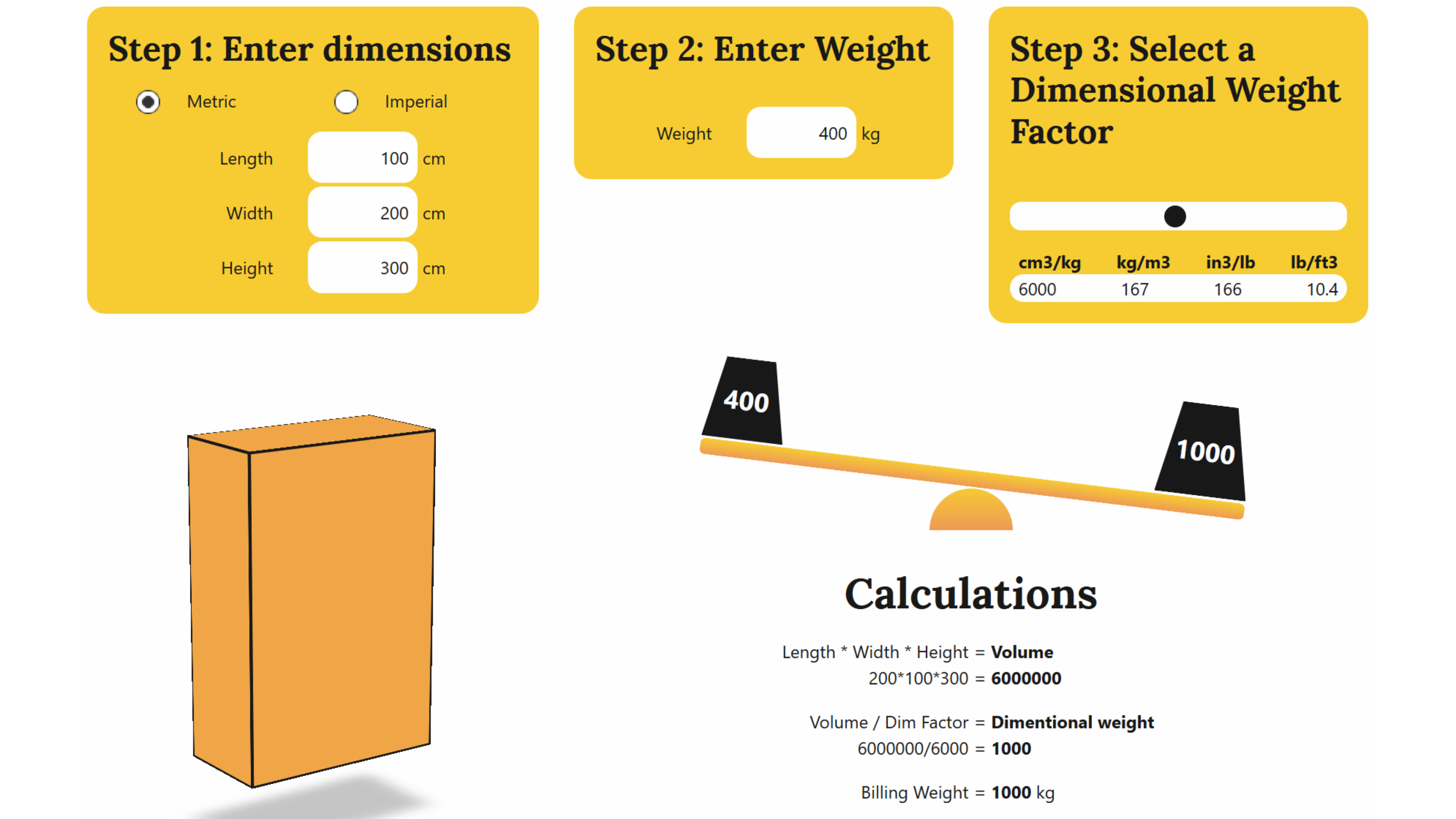# Dimensional Weight - In depth

— 4 minute read

If you are already in the know, you can jump straight to the tool here, if not, please read on.Screen shot of dimesional weight tool

# Dimensional Weight

Dimensional weight. it is a pretty basic concept that is used by transport companies to when calculating freight charges, rather than relying on gross weight alone. This dimensional weight comes into effect often when shipping high volume, low weight items. When charging based on weight, which ever is greater between the dimensional weight, and the actual weight, is used when calculating the charges.

This mechanism is an incentive to shippers of low density products to reduce unnecessary packaging. it can also be a surprise to unsuspecting consumers, who are not aware of how each transport company calculates dimensional weight.

To calculation dimensional weight, first you must calculate volume. For this discussion we will assume that volume is calculated either in cubic centimetres, or cubic inches.

# Calculating Volume

The calculation is very simple:

`Length * Width * Height = Volume`

`volume/dimensional rate factor`

If what you are shipping is not cuboid, you must take the maximum value of each dimension. This means if you are shipping a cylinder, the measurement would be length * diameter * diameter.

# Calculating Dimensional Weight

Dimensional rate factors is where things get a little confusing. They can be expressed in two different ways.

Volume per Weight Unit, or, weight per volume unit. to show a simple example, lets take a look at an example using Cubic CM an kilograms. The example factor we will use is 5000cm3 per 1 kilo.

Lets calculate volume:

`100cm * 100cm * 100cm = 1000000cm3`

to calculate Dimensional weight:

`1000000/5000 = 200kg`

Now, to express the same dimensional rate factor, in terms of weight per volume unit, the number of kilograms per cubic meter is used. a cubic meter just happens to equal 1000000cm3, so from above, we can see that the equivalent expression of 5000cm3 per kilogram, is 200kg per cubic meter. To calculate dimensional weight using this measure, you need the following formula:

`1000000/100000 * 200 = 200kg`

Lets take a slightly more interesting example:

`120*120*120 = 1728000`

`1728000/1000000 * 200 = 345.6kg`

# Dimensional Rate factors

So, with some pretty basic math, we can calculate dimensional weight. One place it gets tricky is working with different carriers, who all seem to have their own way of communicating dimensional rate factors.

Some will use cm3/kg, others kg/m3, and then their is the is the imperial measurements. FedEx for example used 139 cubic inches per pound, or 139in3/lb. This is where my brain fails me. Unfortunately my dad is not always around to do these conversions!

Luckily, there is some hope. The seemingly random in3/lb values are actually based on the metric standards, so 139in3/lb is equivalent to 5000cm3/kg. The same goes for all other examples I was able to find.

cm3/kgkg/m3in3/lblb/ft3
30003338320.8
400025011015.6
500020013912.5
600016716610.4
70001431948.9
80001252217.8
90001112506.9

Taking the above dimensional rate factors, and applying it to an example volume, we get the following result:

`1728000/3000 = 345.6kg`

`1728000/9000= 192kg`

It is literally 3 times lighter. If you are shipping low density goods, know your dim rate factors, and reduce the size of your packaging as much as possible.

To help further articulate dimensional weight, I have built a small tool that can help you calculate dimensional weight easily, which can be found here.

Hope you enjoy playing around with it! If you find it useful, or think others will, please share it too!

# Subscribe to the Flexion Newsletter

Subscribe to get our latest content via email.RSS feed also available.

We will not spam you.

Un-subscribe at any time with a single click.

# Thank you

Thanks for subscribing.